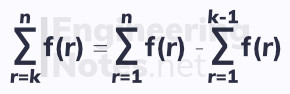#### Notes by Category University Engineering

Electronics*
Mathematics*
Mechanics & Stress Analysis*
Rate these notesNot a fanNot so goodGoodVery goodBrillRate these notes
• A-Level Further Maths

# Series

Sigma notation is used to write series quickly. This describes each term of a series as an equation, where substituting in values for r gives that term in the series.

There are standard sums of series formulae that are used all the time:

### The Sum of Natural Numbers

To find the sum of a series of constant terms, use the formula:To find the sum of the first n natural numbers (positive integers):If the series does not start at r = 1, but at r = k, subtract one series from another, where the first goes from r = 1 to r = k, and the second from r = 1 to r = (k-1):Expressions in sigma notation can be rearranged to simplify complicated series:### The Sum of Squares

The first sum formula above, for constant terms, is linear. The second, for the sum of natural numbers, is quadratic. Therefore, the formula for the sum of square terms is cubic.

To find the sum of the sum of the squares of the first n natural numbers: